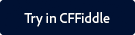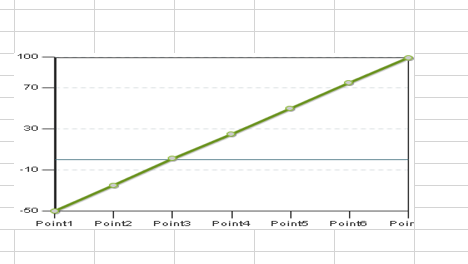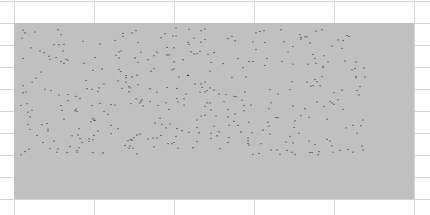Note:

You can find the CFFiddle demo of this function and other spreadsheet functions as part of a project that is shared with you.

Click the button below to launch CFFiddle.To copy the project in your workspace in CFFiddle, follow the steps below:

1. Log in with your Gmail or Facebook credentials.
2. Navigate to the project in the left pane.
3. Once you make some changes in any cfm in the project, a pop up displays asking you to save the project.
4. Give the project a suitable name and click Save.

#### Description

Adds an image to an Excel spreadsheet object.

Nothing

#### Syntax

`SpreadsheetAddImage(spreadsheet,imagePath,anchor,imageData,imageType)`

#### History

ColdFusion 11: Added the function.

#### Parameters

Parameter

Description

spreadsheetObj

The Excel spreadsheet object to which to add the column.

anchor

The image location, as a comma delimited list in either of the following formats:

• startRow,startColumn,endRow,endColumn
• startXPosition,startYPosition,endXPosition,endYPositions,startRow,startColumn,endRow,
endColumn
• The first format specifies only the row and column numbers, the second also specifies the positions in the cell, using pixel X and Y coordinates relative to the cell upper left corner. If you use the first format, the image corner positions within the top left and bottom right cell are 0,0 and ,0,255.

imageData

A ColdFusion image object.

imageFilePath

The absolute path to the image file.

imageType

The image format, one of the following:

• jpg or jpeg
• png
• dib

#### Example 1

The following example creates a PNG format chart, puts it in a new spreadsheet between (5,5) and (12,10), and writes the spreadsheet object to a file.

```<cfchart format="png" scalefrom="-50" scaleTo="100" gridlines="5" name="myChart">
<cfchartseries type="line">
<cfchartdata item="Point1" value="-50">
<cfchartdata item="Point2" value="-25">
<cfchartdata item="Point3" value="1">
<cfchartdata item="Point4" value="25">
<cfchartdata item="Point5" value="50">
<cfchartdata item="Point6" value="75">
<cfchartdata item="Point7" value="99">
</cfchartseries>
</cfchart>

<cfscript>
theDir=GetDirectoryFromPath(GetCurrentTemplatePath());
SpreadsheetObj=SpreadsheetNew();
SpreadsheetAddImage(SpreadsheetObj,myChart,"png","5,5,12,10");
SpreadSheetWrite(SpreadsheetObj,"#theDir#imagesheet.xls",true);
</cfscript>```

#### OutputLine output

#### Example 2

The following example creates a PNG format series of random points on a 400x400 image and writes the image on a spreadsheet.

```<cfscript>
// Create a ColdFusion image of points
myImage=ImageNew("",400,400,"rgb","lightgray");
for (i=10;i<400;i++){
ImageSetDrawingColor(myImage,"black");
x=randRange(5,350);
y=randRange(10,300);
ImageDrawPoint(myImage,x,y);
}
// Add points to spreadsheet object and write to file
theDir=GetDirectoryFromPath(GetCurrentTemplatePath());
SpreadsheetObj=SpreadsheetNew();
SpreadsheetAddImage(SpreadsheetObj,myImage,"png","5,5,12,10");
SpreadSheetWrite(SpreadsheetObj,"#theDir#imagesheet1.xls",true);
</cfscript>```

#### OutputPoint outputTwitter™ and Facebook posts are not covered under the terms of Creative Commons.